#### You may also like### Prompt Cards

These two group activities use mathematical reasoning - one is numerical, one geometric.### Consecutive Numbers

An investigation involving adding and subtracting sets of consecutive numbers. Lots to find out, lots to explore.### Exploring Wild & Wonderful Number Patterns

EWWNP means Exploring Wild and Wonderful Number Patterns Created by Yourself! Investigate what happens if we create number patterns using some simple rules.

# Number Squares

##### Age 5 to 11Challenge Level

This challenge is a bit different to my usual ones. I used it many years ago when I wanted some quite young children to do lots of adding in a more fun way.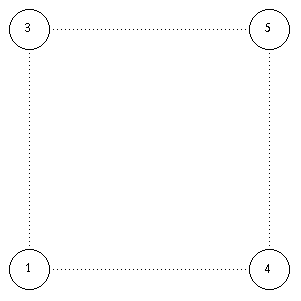You start with four numbers at the corners of a square. Then you add up the numbers at the two ends of each side and put the total in the middle of that side. So in my example 3 + 5 = 8, 5 + 4 = 9, 3 + 1 = 4, 1 + 4 = 5.These four new answers give us the corners of a new square. The corners are 8, 9, 5 and 4.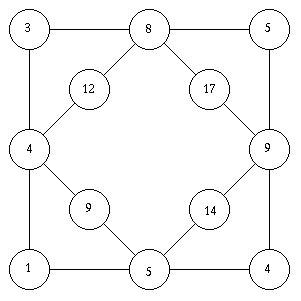These four new numbers are added up and the answers put in the centre of the edges of this new square. And so on and so on.

The diagram gets more and more complicated, growing as shown below:-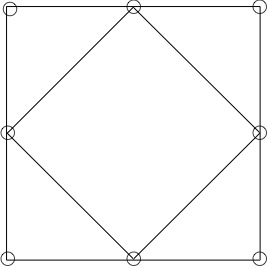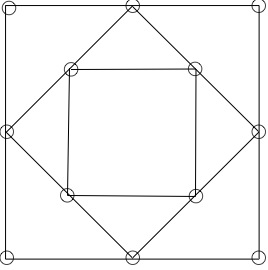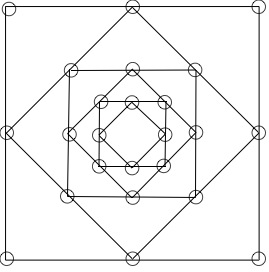There is not much more to say, apart from have a go yourself. Use any starting numbers at the corners. Can you estimate what the size of the last four numbers will be?

What would happen if you used different shapes, for example pentagons or hexagons?

What would happen if you used subtraction, always taking the smaller from the bigger?

What would happen if you multiplied? Divided? What ...??